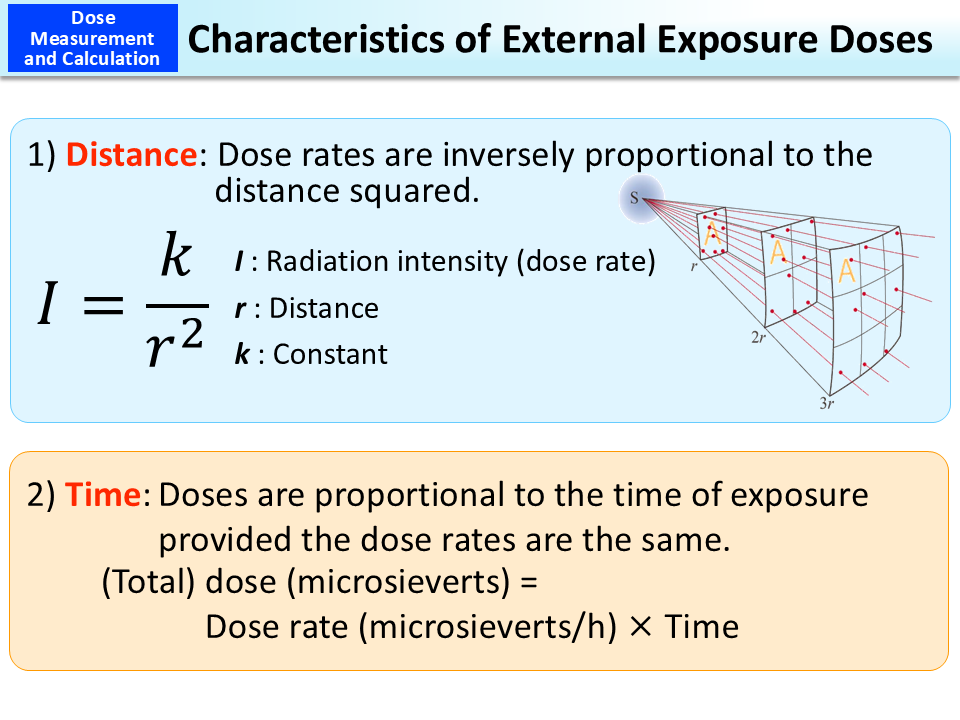BOOKLET to Provide Basic Information Regarding Health Effects of Radiation (3rd Edition)

# Characteristics of External Exposure DosesThe intensity of radiation (dose rate) is strong (large) when the source of radiation (radiation source) is close, and it gets weaker (smaller) as the distance increases, even if the amount of radioactive materials remains the same. When the radioactive materials are located only in one place (point source), the dose rate becomes smaller in inverse proportion to the distance squared. Dose rates also decrease due to atmospheric influence, etc.

When radioactive materials are evenly distributed on a broad plain surface, the formula to express the relationship between the distance and the dose rate is rather complicated, but as in the case of a point source, the higher it is from the ground surface, the lower the dose rate is. However, radioactive materials are not evenly distributed in reality and a plain surface is not necessarily smooth, and also owing to attenuation of radiation in the air or other reasons, the dose rate does not always match the value obtained from the relational expression.

Calculation of external exposure doses is based not on the radioactivity intensity (becquerels) but on the amount of radiation (grays or sieverts) the human body is exposed to.

If the dose rate is constant, the total exposure dose can be calculated by multiplying the dose rate by the time of exposure to radiation.

• Included in this reference material on March 31, 2013
• Updated on March 31, 2019